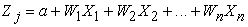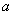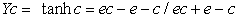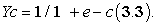﻿ Using Discriminant Analysis and Artificial Neural Network Models for Classification and Prediction of Fertility Status of Friesian CattlePublications are Open
Access in this journal
Article Versions
Export Article
• Normal Style
• MLA Style
• APA Style
• Chicago Style
Research Article
Open Access Peer-reviewed

### Using Discriminant Analysis and Artificial Neural Network Models for Classification and Prediction of Fertility Status of Friesian Cattle

Eman A. Abo Elfadl , Fatma D. M. Abdallah
American Journal of Applied Mathematics and Statistics. 2017, 5(3), 90-94. DOI: 10.12691/ajams-5-3-1
Published online: August 05, 2017

### Abstract

Background & objectives: This study was undertaken to compare the accuracies of Discriminant analysis model (DA) and Artificial neural networks model (ANN) for classification and prediction of Friesian cattle fertility status by using its reproductive traits. Methods: Data was collected through field survey of 2843 animal records of Friesian breed belongs to El Dakhalia province farms, Egypt. Data was covering the period extended from 2010 to 2013. The samples of dairy production sectors were selected randomly. Data was collected from valid farm records or the structured questionnaires established by the researcher. Results: The results of classification accuracy indicated that the artificial neural network (ANN) model is more efficient than the discriminant analysis (DA) model in expressing overall classification accuracy and accuracies of correctly classified cases of fertility status for Friesian cattle. The results showed that The ANN models had shown the highest classification accuracy (93.6%) for year (2010) while, it was (79.9%) for DA. The comparison of overall classification accuracies clearly favored the supremacy of ANN over DA. The results also were confirmed by the areas under Receiver Operating Characteristic Curves (ROC) captured by ANN and DA. ROC curves are used mainly for comparing different discriminating rates. Areas under ROC curves were higher in case of ANN models across the different years compared to DA models. The differences in accuracies were also significant at 5% level of significance with p-value 0.005 by using Paired Sample t-test. From all of the above we can conclude that artificial neural network model was more accurate in prediction and classification of fertility status than a traditional statistical model (Discriminant analysis).

### 1. Introduction

Discriminant Analysis is mainly used for classifying data into two categories or more. The discriminating function is the linear combination of the two (or more) independent variables that will discriminate between the different categories in the grouping variable. It is performed by calculating the weights for each independent variable to maximize the differences between the groups that are between-group variance relative to the within-group variance 1.

In some case, linear discriminants are insufficient for discrimination process and for minimizing the error. For this reason, Multilayer neural networks can do well and make classification in the same manner as linear discriminant and overcome all problems which may occur.

Artificial neural network (ANN) or connectionist systems are a computational model made up of a number of simple, highly interconnected processing elements, which process information by their dynamic state response to external inputs. It is mainly used in computer science and other research disciplines, which is based on a large collection of simple neural units (artificial neurons) 2.

The multi-layer perceptron Neural Network is a standard layered Neural Network type with a linear accumulation and a sigmoid transfer function. Usually the network consists of an input layer, receiving the measurement vector x, a hidden layer and an output layer of units (neurons). In this configuration each unit of the hidden layer realises a hyper plane dividing the input space into two semi spaces. By combining such semis paces the units of the output layer are able to construct any polygonal partition of the input space 3.

Artificial neural networks (ANN) represent new approach for data prediction and classification. Recently, many publications had applied artificial neural networks to natural resources topics include: biophysical interactions complex modeling for resource planning applications 4; generating terrain textures from a digital elevation model 5; tree survival probabilities model 6 and 7 who used geographic information systems (GIS) in developing computer-aided visualization of proposed road networks.

The present study was delineated to compare the accuracies of the most popular technique used for data prediction and classification that is discriminant analysis with a comparatively newer one that is Artificial Neural Network using dairy animal data. Data of reproductive traits affection fertility status of the animals were entered as (independent variables) while fertility status (healthy or diseased) was entered as (dependent one) to determine the most efficient technique for data classification and prediction.

### 2. Material and Methods

2.1. Source of Data

This study was carried out through field survey of 2843 animal records of Friesian breed belongs to El Dakhalia province farms, Egypt. Data was covering the period extended from 2010 to 2013 depending on random samples of dairy production sectors. Data was collected from valid farm record or the structured questionnaires established by the researcher in accordance with objectives of this study and were admitted to the dairy holders and managers during the time of interview.

2.2. Research Hypothesis

Ho: There is no statistically significant difference in the classification accuracies of fertility status by Artificial Neural Network model and Discriminant Analysis.

HA: There is statistically significant difference in the classification accuracies of fertility status by Artificial Neural Network model and Discriminant Analysis.

2.3. Studied Variables

• The raw data were collected from reproduction records which include:

(Calving interval /day): It is the period of time between two successive parturitions.

(Days open /day): It is the period of time between parturition and the next conception.

(Days in milk or lactation length): It is the average lactation length of milk per day.

(Parity) =No. of lactation season.

(Days to first insemination / day): It is the period from calving till first insemination.

(Dry period): period from drying till next parturition /day.

• Animals of one or more reproductive diseases were coded as one (1= diseased) and healthy animals were coded as zero (0 = normal).

2.4. Statistical Analysis
2.4.1. Discriminant Analysis

Discriminant analysis is used for classifying data into two more than two categories. The discriminating function is the linear combination of the two (or more) independent variables that will discriminate best between the categories of the grouping variable. It is achieved by calculating the weights for each independent variable to maximize the differences between the groups that are between-group variance relative to the within-group variance. In this study, DA was carried out to check the significance of reproductive trait (independent variables) to predict the fertility status of the animal (dependent variable). All reproductive traits (independent variable) including: (calving interval, days open, days in milk, parity, days to first insemination and dry period) and fertility status (dependent variable) which was coded as following (0= normal, 1= diseased) were entered in DA model using statistical package (SPSS, version 20.0) then the weights of Discriminant analysis were calculated from an equation much like that seen in multiple regressions. It takes the following form as mentioned by Hair et al (2009).(1)

Where,

Zjk = discriminant Z score of discriminant function j,= intercept

Wi = discriminant weight for independent variable, I,

Xik = independent variable.

2.4.2. Artificial Neural Network

Network computation consists mainly of dense mesh of computing nodes and connections. They operate collectively and simultaneously on most or all data and inputs. The basic processing elements of neural networks are called artificial neurons, or simply neurons. Neurons perform as summing and nonlinear mapping junctions.

Herein, ANN was carried out to check the significance of reproductive trait (independent variables) to predict the fertility status of the animal (dependent variable) which was coded as follows ( 0= normal animal, 1= diseased) all reproductive traits (independent variable) including: (calving interval, days open, days in milk, parity, days to first insemination and dry period) and fertility status (dependent variable) were entered in ANN model using statistical package (SPSS, version 20.0) and then the connection strength, is expressed as a numerical value called a weight, which can be modified. A typical Neural Network diagram of data set for Friesian cattle throughout 2010and 2011 were shown in Figure1.

View option
• Figure 1. Artificial neural networks for year 2010 and 2011 as an example of ANN

Hyperbolic Tangent function was used as activation function for hidden layer. Range of nodes in hidden layers was set as 1 to 50, Batch Training was used for training network (vi) Scaled Conjugate Method was used as Optimization algorithm. Initial Lambda was set as 0.0000005. Initial Sigma was set as 0.00005, Interval centre was set as 0.00 Interval offset was set as ± 0.50. Minimum Relative change in Training Error was set as 0.0001 1.

Hyperbolic Tangent function has the following form:(2)

Where, c is the input from previous nodes. Y(c) takes real-value arguments and transforms them to the range (-1, +1). Sigmoid function has the following form:(3)

Y(c) takes real-value arguments and transforms them to the range (0, 1).

Comparison of classification results produced by models was done with the help of ROC curves and the classification accuracies of both models results had been compared by Paired Sample t-test after testing these accuracies for the normality by using One Sample Kolmogorov Smirnov test.

### 3. Results

The result in Table 1 had showed the Overall Classification Accuracies represented in percentages for Artificial Neural Network (ANN) and Discriminant Analysis (DA). The last column showed difference in accuracies between two models:

The ANN models had shown the highest classification accuracy for year 2010 (93.6%) and the lowest for year 2013 (85.0%). while, DA had shown the highest classification accuracy for year 2011 (89.4%) and the lowest also for year 2013(74.0%). The comparison of overall classification accuracies clearly favored the supremacy of ANN over DA. Especially, for year 2010 as the difference between two models in the accuracy of classification was +13.

It was also founded that the differences in the accuracies between two models were statistically significant with (p-value = 0.041) by using Paired Sample t-test after testing this accuracies for normality by using One Sample Kolmogorov Smirnov test and p-values were 0.859 and 0.326 respectively.

The accuracies of correctly classified cases of normal and diseased animal also were compared; the highest accuracy of classification for normal cases was achieved by DA (99.7%). The ANN models had shown the highest classification accuracy of normal cases for year 2012 (97.5%) and the lowest for year 2010 (92.2%), while the DA models had shown the highest classification accuracy of normal cases for year 2010 (99.7%) and lowest for year 2012 (77.9%). On the other hand, the highest accuracy of classification for diseased cases was achieved by ANN (94.2%) and The ANN models had shown the highest classification accuracy of diseased cases for year 2010 (94.2%) and the lowest for year 2012 (62.6%), while the DA models had shown highest classification accuracy of diseased for year 2013 (86.0%) and lowest for year 2010 (71.4%) as showed in Table 2. The differences in accuracies of correctly classified cases for both control and diseased cases were not significant at 5% level of significance by Paired Sample t-test.

The results were also confirmed by the areas under Receiver Operating Characteristic Curves (ROC) captured by ANN and DA. ROC curves are plotted against (1-specificity) on X-axis and sensitivity on Y-axis for a range of cut-offs. Herein, ROC curves were used mainly for comparing different discriminating rates.

As showed in Table 3, areas under ROC curves were higher in case of ANN models across the different years as compare to DA models. The differences between these areas were founded significant with p-value 0.005 by using Paired Sample t-test. The Overall classification results and Areas under ROC curves for ANN and DA models were significantly better for ANN models.

The important predictors for the classification process by ANN were days open and days to first insemination with large coefficients (0.382, 0.336, 0.789 and 0.605), respectively, while for DA, dry period, days open and days to first insemination (0.886, 0.640,0.295 and 0.733) seemed to be the most important ones as shown in Table 4.

### 4. Discussion

In the present study, the results had shown that the ANN model was more efficient than the DA model in the prediction and classification of fertility status of Friesian dairy cattle. This is may be due to the assumptions associated with DA. In discriminant analysis, we assumed that the distribution of both dependent and independent is normal. But In fact, some variables which had been used in this study were not normally distributed. So, these factors had a great effect on the DA results. These results had been confirmed by many studies which reported that DA is very robust to data of different type. As 9 which states that:" In applied research, data are seldom compatible with the underlying assumptions needed to perform statistical inferences".

It also founded that the ANN model had performed higher classification accuracies than the DA, either in cases of only quantitative independent variables or both qualitative and quantitative independent variables were used. This may be due to the fact that the artificial neural network not affected by the type of distributions for the variables used 10.

In a previous work, the results of classification accuracy indicated that the ANN model is more efficient than the DA model in expressing overall classification accuracy, accuracies of correctly classified cases. The results had showed that The ANN models shown the highest classification accuracy (93.6%) for year (2010) while, it was (79.9%) for DA. The comparison of overall classification accuracies clearly favored the supremacy of ANN over DA. Especially, for year 2010 as the difference between two models in the accuracy of classification was +13. The differences were found significant at 5% level of significance with p-value (0.041) by using paired t-test. These results also were confirmed by the areas under Receiver Operating Characteristic Curves (ROC) captured by ANN and DA. ROC curves were used mainly for comparing different discriminating rates. Areas under ROC curves were higher in case of ANN models across the different years as compare to DA models. The differences were found significant at 5% level of significance with p-value 0.005 by using Paired Sample t-test. The Overall classification results and Areas under ROC curves for ANN and DA models were significantly better for ANN models. Furthermore, there was no problem in this study concerning sample size because very large number observations were available for analysis and hence all data sets contained adequate numbers of observations. This fact may pose a problem for many researchers as reported by 11.

We had also described an earlier attempt to predict fertility status of Friesian cattle by using Discriminant Analysis (DA) and the Artificial Neural Network (ANN). And the finding were In contrast to the findings obtained by 11 which reported that both the ANN and DA methods performed rather poorly in the classification process of the data.

### 5. Conclusion

The Discriminant Analysis (DA) and the Artificial Neural Network (ANN) had showed comparable results suggesting that a linear discrimination of the input is not sufficient model for Classification and prediction of group membership for the studied data. The ANN model is more efficient than the DA model in expressing overall classification accuracy, accuracies of correctly classified cases for fertility status of the animals. It may be useful to apply Neural Networks analysis if the independent variables were used for prediction and classification process are not normally distributed.

### References

  Iyer E., Murti V., K. and Arora, V, “Comparison of Artificial Neural Network and Multiple Discriminant Analysis Models for Bankruptcy Prediction in India”. IJAPRR International Peer Reviewed Refereed Journal, 3(1):12-21, 2016. In article  Hecht-Nielsen R, “Cogent confabulation”. Neural Networks, 18: 111-115, 2005. In article View Article  PubMed  Schumann, A, “Neural Networks versus Statistics: A Comparing Study OF Their Classification Performance on Well Log Data”. Free University of Berlin, Geoinformatics. Germany, 12249 Berlin, Malteserstr, 1997, 74-100. In article View Article  Gimblett, R.H. and G. L. Ball, “Neural network architectures for monitoring and simulating changes in forest resources management”. AI Applications, 9: 103-123, 1995. In article View Article  Alvarez, S, “Generation of terrain textures using neural networks”. Unpublished master thesis. Department of Computer Science, Colorado State University, 1995, 44. In article View Article  Guan, B. T. and G. Gertner, “Modeling individual tree survival probability with a random optimization procedure”: An artificial neural network approach. AI Application, 9: 39-52, 1995. In article View Article  Harvey, W., Dean, D.J, “Computer-aided visualization of proposed road networks using GIS and neural networks”. In: First Southern Forestry GIS Conference. G.J. Arthaud, W.C. Hubbard (Eds.). University of Georgia, Athens, GA, 1996, 416-417. In article  Hair J., Black W. C., Babin B. J., Anderson, R. E., and Tatham, R. L, “Multivariate Data Analysis”, 6th edition, Logistic Regression: Regression with a Binary Dependent Variable, 2009, 379. In article PubMed  Yoon, Y., Swales, G., Margavio, T.M., “A comparison of discriminant analysis versus artificial neural networks”. J. Oper. Res. Soc., 44 (1): 51-60.1993. In article View Article  Marzban, C., Paik, H., Stumpf, G, “Neural networks versus Gaussian discriminant analysis”. AI Appl., 11 (1): 49-58.1997. In article View Article  Blackard, J.A., Dean, D.J., “Evaluating integrated artificial neural network and GIS techniques for predicting likely vegetative cover types”. In: First Southern Forestry GIS Conference. G.J. Arthaud, W.C. Hubbard (Eds.). University of Georgia, Athens, GA, 1996, 416-417. In articleThis work is licensed under a Creative Commons Attribution 4.0 International License. To view a copy of this license, visit http://creativecommons.org/licenses/by/4.0/